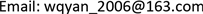﻿ 模糊综合评价法在工程项目风险分析中的应用 Application of Fuzzy Comprehensive Evaluation Method in Project Risk Analysis

Service Science and Management
Vol. 08  No. 03 ( 2019 ), Article ID: 30516 , 6 pages
10.12677/SSEM.2019.83014

Application of Fuzzy Comprehensive Evaluation Method in Project Risk Analysis

Qingyuan Wang, Wen Guo

Institute of Underground Space for Stability and Support of Surrounding Rock, Heze University, Heze ShandongReceived: May 8th, 2019; accepted: May 22nd, 2019; published: May 29th, 2019ABSTRACT

With the increase of project scale, project risks become more and more, which is more difficult to predict and evaluate. Scientific and systematic risk management and prediction are conducive to improving the quality of decision-making, expanding the operation efficiency of the project and bringing a stable environment for the project. This paper introduces the fuzzy comprehensive evaluation model and analyzes the risk of the creative building by establishing the fuzzy comprehensive evaluation model. The analysis results show that the risk level of the creative building is between V1 and V2, and the overall risk level of the project is low and the safety is high.

Keywords:Risk Management, Fuzzy Comprehensive Evaluation Method, Engineering ProjectCopyright © 2019 by author(s) and Hans Publishers Inc.1. 引言

2. 工程项目风险模糊综合评价模型

2.1. 建立评语集与因素集

$U=\left\{{U}_{1},{U}_{2},\cdots ,{U}_{n}\right\}$ (1)

${U}_{n}=\left\{{U}_{ni},{U}_{n2},\cdots ,{U}_{nm}\right\}$ (2)

2.2. 评价隶属矩阵的建立

${R}_{ij}=\left({r}_{ij1},{r}_{ij2},\cdots ,{r}_{ijn}\right)$ (3)

${r}_{ijn}\left(n=1,2,\cdots ,s\right)$ 的取值方法为：把收集好的专家意见进行统计，统计专家对因素集U的评价个数，如专家对Uij有rijn个Vn等级评价，那么有：

${r}_{ijn}={V}_{ijn}/\sum {V}_{ijl}\left(\sum {V}_{ijl}={V}_{ij1}+{V}_{ij2}+\cdots +{V}_{ijn}\right)$ (4)

${R}_{n}={\left({R}_{n1},{R}_{n2},\cdots ,{R}_{nm}\right)}^{\text{T}}$ (5)

${R}_{n}=\left[\begin{array}{cccc}{r}_{11n}& {r}_{12n}& \cdots & {r}_{1cn}\\ {r}_{21n}& {r}_{22n}& \cdots & {r}_{2cn}\\ ⋮& ⋮& \ddots & ⋮\\ {r}_{k1n}& {r}_{k2n}& \cdots & {r}_{kcn}\end{array}\right]$ (6)

2.3. 建立各个风险的权重级

2.4. 一致性检验

$CI=\left({\lambda }_{\mathrm{max}}-n\right)/\left(n-1\right)$ (7)

$CR=CI/RI$ (8)

${B}_{i}=\left({b}_{i1},{b}_{i2},\cdots ,{b}_{in}\right)$ (9)

2.5. 模糊综合评价

${B}_{i}={A}_{i}\cdot {R}_{i}$ (10)

$B=A\cdot R=\left({\lambda }_{1},\cdots ,{\lambda }_{n}\right)\cdot R$ (11)

$\stackrel{¯}{\lambda }={\lambda }_{i}/\underset{i=1}{\overset{n}{\sum }}{\lambda }_{i}$ (12)

3. 案例分析

3.1. 项目概述

3.2. 风险因素集分析

3.3. 风险等级的隶属度Rij

${R}_{r1}=\left[\begin{array}{cccc}0.8& 0.2& 0& 0\\ 0.4& 0.4& 0.2& 0\\ 0.2& 0.4& 0.2& 0.2\\ 0.4& 0.2& 0.2& 0.2\end{array}\right]$

${R}_{r2}=\left[\begin{array}{cccc}0& 0.4& 0.4& 0.2\\ 0.2& 0.2& 0.4& 0.2\\ 0& 0.6& 0.2& 0.2\\ 0.2& 0.4& 0.4& 0\end{array}\right]$

${R}_{r3}=\left[\begin{array}{cccc}0.2& 0& 0.6& 0.2\\ 0.4& 0.2& 0.2& 0.2\\ 0.6& 0.2& 0.2& 0\\ 0.4& 0.4& 0.2& 0\end{array}\right]$

3.4. 一致性检验

3.5. 模糊综合评价

1) 一级模糊综合评价

${B}_{1}={A}_{1}\cdot {R}_{r1}=\left[\begin{array}{cccc}0.3804& 0.2822& 0.1918& 0.1454\end{array}\right]$

${B}_{2}={A}_{2}\cdot {R}_{r2}=\left[\begin{array}{cccc}0.1008& 0.4647& 0.3098& 0.1454\end{array}\right]$

${B}_{3}={A}_{3}\cdot {R}_{r3}=\left[\begin{array}{cccc}0.4681& 0.2483& 0.2152& 0.0953\end{array}\right]$

2) 二级模糊综合评价

$B=A\cdot {R}_{r}=\left[\begin{array}{cccc}0.3951& 0.2832& 0.2204& 0.1044\end{array}\right]$

$B=\left[\begin{array}{cccc}0.3939& 0.2823& 0.2197& 0.1041\end{array}\right]$

4. 结论

Application of Fuzzy Comprehensive Evaluation Method in Project Risk Analysis[J]. 服务科学和管理, 2019, 08(03): 96-101. https://doi.org/10.12677/SSEM.2019.83014

1. 1. 李开孟. 工程项目风险分析评价理论方法及应用[M]. 北京: 中国电力出版社, 2017: 1-13.

2. 2. 乔永峰, 方圆，褚亚文. VAR在项目风险分析与评价中的应用[J]. 内蒙古财经学院学报, 2007(5): 61-63.

3. 3. 高辉. 基于风险分析的房地产项目决策与对策研究[D]: [博士学位论文]. 西安: 西安建筑科技大学, 2005.

4. 4. 王莉. 模糊分析法在工程项目风险分析中的应用[J]. 物流工程与管理, 2011, 33(6): 161-163.

5. 5. 袁杰, 梁雪春. 层次分析法中判断矩阵的一致性改进[J]. 统计与决策, 2014(12): 15-17.

6. 6. 刘林. 应用模糊数学[M]. 北京: 清华大学出版社, 2005.